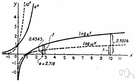# exponential function

(redirected from Exp (programming))
Also found in: Thesaurus, Encyclopedia.
ThesaurusAntonymsRelated WordsSynonymsLegend:
 Noun 1exponential function - a function in which an independent variable appears as an exponentexponentialfunction, mapping, mathematical function, single-valued function, map - (mathematics) a mathematical relation such that each element of a given set (the domain of the function) is associated with an element of another set (the range of the function)
Based on WordNet 3.0, Farlex clipart collection. © 2003-2012 Princeton University, Farlex Inc.
Site: Follow: Share:
Open / Close